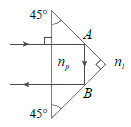Problem: Consider a prism with the shape shown in the diagram. Its index of refraction is labeled by np, and it is submerged in a special liquid which has an index of refraction n ℓ, where the difference np − nℓ = 0.59. The light ray is perpendicularly incident from the liquid into the prism as shown in the diagram. Notice that the incident angle at both points A and B is 45°. Find the index of refraction of the prism for a critical angle of 45°.

FREE Expert Solution
91% (401 ratings)
Problem Details

Consider a prism with the shape shown in the diagram. Its index of refraction is labeled by np, and it is submerged in a special liquid which has an index of refraction n , where the difference np − n = 0.59. The light ray is perpendicularly incident from the liquid into the prism as shown in the diagram. Notice that the incident angle at both points A and B is 45°. Find the index of refraction of the prism for a critical angle of 45°.